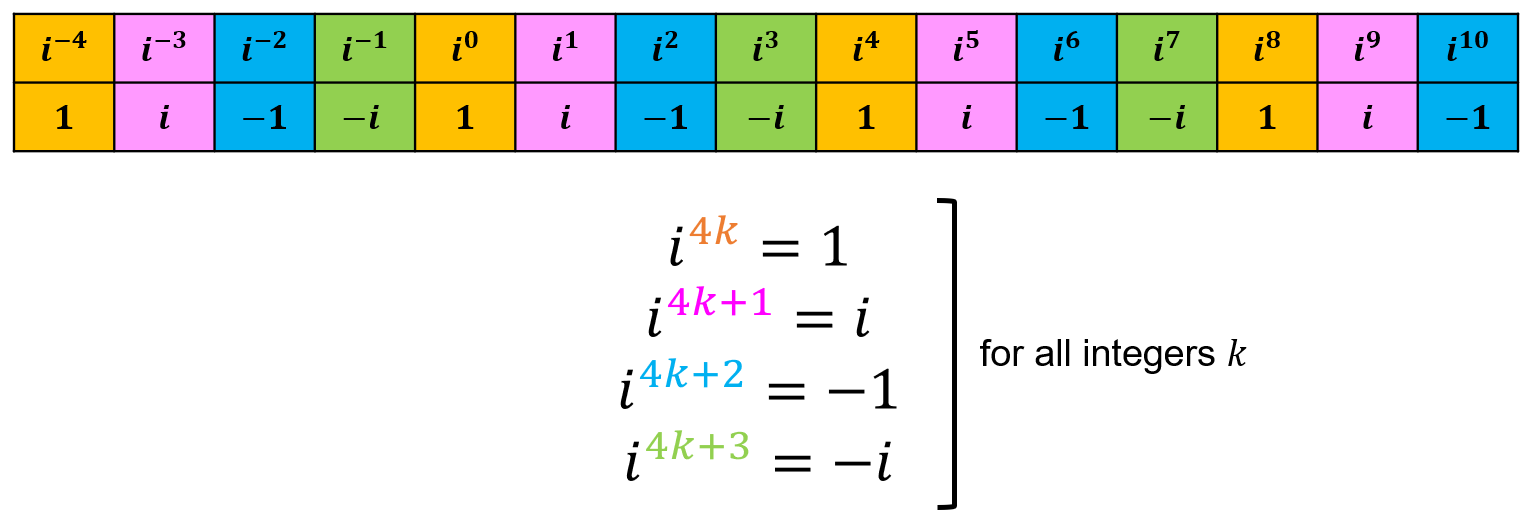# Complex Numbers

## Powers of i

By definition, we know that $$i=\sqrt{-1}$$ and thus $$i^2=-1$$ and we also have that $$i^0=1$$ (since any number to the exponent of $$0$$ is equal to 1). But what if we wanted to find $$i^{2044}$$?

If we list out the first few positive integral powers of $$i$$, we can see a pattern starting to form:

$$i^3=-i,\ i^4=1, \ i^5=i,\ i^6=-1, \ i^7=-i, \ i^8=1, \ i^9=i, \ i^{10}=-1, \ ...$$

A similar pattern for the first few negative integral powers of $$i$$ occurs as well:

$$i^{-1}=\frac{1}{i}=-i$$,

$$i^{-2}=\frac{1}{i^2}=\frac{1}{-1}=-1$$,

$$i^{-3}=i^{-2}\cdot i^{-1}=-1(-i)=i$$,

$$i^{-4}=i^{-2}\cdot i^{-2}=-1(-1)=1$$, ...

So we can summarize these observations as follows:And now we have a quick way to determine larger integral powers of $$i$$!

So we can apply this to our previous example:

Example: Evaluate $$i^{2044}$$.

Since 2044 is divisible by 4 (i.e. $$4k=2044$$ when $$k=511$$), $$i^{2044}=1$$.

## Evaluating Integral Powers

Now what if we wanted to evaluate integral powers of complex numbers with both a real and imaginary part?

To do so, we first have to introduce DeMoivre's Formula, which will allow us to raise complex numbers to any exponent when its in polar form (to review rectangular and polar form conversion see this page):

$$(cos(x)+isin(x))^n=cos(nx)+isin(nx)$$,

for any integer $$n$$ and real number $$x$$.

We can apply this to evaluating integral powers of complex numbers (in polar form) as follows:

$$(r(cos(\theta)+isin(\theta))^n$$

$$=r^n(cos(\theta)+isin(\theta))^n$$

$$=r^n(cos(n\theta)+isin(n\theta))$$

Example: Evaluate $$(-3-3i)^4$$.

Solution:

1. Notice that $$-3-3i$$ is in rectangular form, so we must convert it to polar form before applying the formula. We converted this number as an example on this page and found that $$-3-3i$$ in polar form is $$3\sqrt{2}(cos(\frac{5\pi}{4})+isin(\frac{5\pi}{4}))$$.

2. Identify the modulus and argument of the complex number. In this case, we have:

$$r=3\sqrt{2}, \ \theta=\frac{5\pi}{4}$$

3. Finally, we can substitute these values into the above formula and simplify:

$$(3\sqrt{2}(cos(\frac{5\pi}{4})+isin(\frac{5\pi}{4})))^4$$

$$=(3\sqrt{2})^4(cos(\frac{5\pi}{4})+isin(\frac{5\pi}{4}))^4$$

$$=3^4(\sqrt{2})^4(cos(4(\frac{5\pi}{4}))+isin(4(\frac{5\pi}{4}))$$

$$=324(cos(5\pi)+isin(5\pi))$$

Notice that $$cos(5\pi)=-1$$ and $$sin(5\pi)=0$$. So, we can further simplify this to get $$(-3-3i)^4=-324$$.